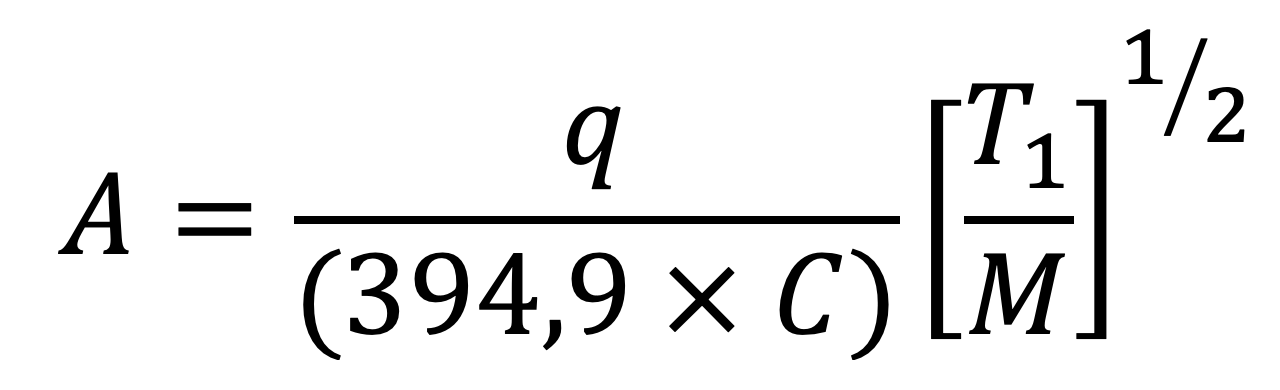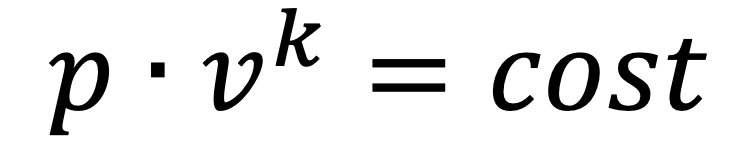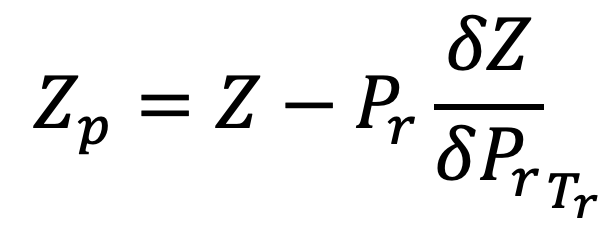# The importance of  k  for safety valve

##### by Rentato Pintabona and Panio Colacicco

The sizing of safety valves designed to discharge gases or vapours, according to lspesl Collection “E”, requires knowledge of the isoentropic exponent k at discharge conditions.

Careless application of the lspesl Collection “E” chapter “E.1”, concerning the sizing of safety valves, can lead to an overestimation of the discharge capacity of valves and rupture discs.

### This article gives some guidelines to estimate the value of k for real gases and highlights the mistake by considering k equal to the ratio of specific heats Cp/Cv

A first and gross mistake to be avoided is to use the formula in Collection ‘E’, valid for gases or vapours, in situations where a two-phase discharge of liquid and gas/vapour takes place. In such cases, in fact, the calculated diameters will undoubtedly be undersized compared to the real need.
A second error, which in many cases can lead to the undersizing the safety system, is to give the isoentropic exponent k the value of the Cp/Cv ratio. While the first point will be the subject of a series of subsequent articles, here we would like to give some useful hints for calculating the isoentropic exponent and show, in concrete cases, the size of the error that can be made.

## Isoentropic outflow through a nozzle

The formula  that is used in the collection “E”, as well as in other Italian  and foreign  standards, for the calculation of safety valves that must discharge gases or vapours, is that of the isoentropic outflow through a nozzle under critical jump conditions, which for an ideal gas is:where the expansion coefficient C is given by:being k the exponent of the isoentropic expansion equation:FluidP1 (bar)T1 (°C)q’ (kg/h)q (kg/h)(q’/q) x 100
Methane125014721466100.4
Methane2320023142267102.1
Propane1210022612181103.7
Hexane1217830992740113.1
Hexane2322065195111127.5
Heptane1221532322821114.4

### q’= flow rate calculated with k = Cp/Cv (20 °C, 1 atm) q = flow rate calculated with k = (Cp/Cv) • (Z/Zp)

By introducing the experimental coefficient k of safety valve outflow, which globally considers the real outflow performance of the valve, a safety coefficient of 0.9 and the compressibility factor Z1 for the real fluid, we arrive at the formulation of the collection “E”:The isoentropic exponent k can be expressed as:

For an ideal gas, for which P x V / R x T =1 , it is demonstrated that k is equal to the ratio Cp/Cv between the specific heats at constant pressure and volume.

For a real gas, k can be expressed (see Appendix B) by:

where Z is the compressibility factor defined by Z=P x V / R x T and Zp is the “derived compressibility factor”. When applying formula , according to collection “E”, the values of Cp/Cv, Z and Zp must be evaluated at discharge conditions P1 and T1.

The derived compressibility factor Zp is defined in formula  as:The compressibility factor Z can be expressed as:

and similarly,  can be expressed as:

where the values of Z^0, Z^1, Zp^0, Zp^1 are tabulated in Appendix A as a function of Pr and Tr.

In  and , Ω is Pitzer’s acentric factor defined by:

Where Pr^SAT is the reduced vapour pressure corresponding to a reduced temperature value Tr=T/Tc=0,7. Appendix A shows the Ω values of some fluids. Z e Zp can also be derived directly from an analytical equation of state.

## A numerical example

Turning to a numerical example, suppose we need to calculate the discharge capacity of a safety valve under the following conditions:

 Fluid n-Butano Physical state superheated vapour Molecular mass M 58,119 Set pressure P 19,78 bar Overpressure 10% Fluid temperature T 400 K Efflux coefficient 0,9 Orifice diameter Do 100 mm

## 147060

Applying formula , with calculation of the flow rate

## 174848

Applying formula , using the value of Cp/Cv at 1 atm and 20 °C

# OVER 20%

To give an idea, the following table shows the flow rates of an 18-mm orifice for other saturated hydrocarbons, calculated in the two cases. The calculations were performed with specially developed software.

FluidP1 (bar)T1 (°C)q’ (kg/h)q (kg/h)(q’/q) x 100
Methane125014721466100.4
Methane2320023142267102.1
Propane1210022612181103.7
Hexane1217830992740113.1
Hexane2322065195111127.5
Heptane1221532322821114.4

The software does not use formulae   but, starting from the modified Redlich and Kwong equation of state, calculates the value of the isoentropic exponent using thermodynamic correlations.